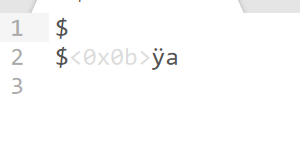# Unusual behaviour with character pointers

• Difficulty Level : Easy
• Last Updated : 03 Nov, 2020

In C++, cout shows different printing behaviour with character pointers/arrays unlike pointers/arrays of other data types. So this article will firstly explain how cout behaves differently with character pointers, and then the reason and the working mechanism behind it will be discussed.

Example 1:

## C++

 `// C++ program to illustrate difference``// between behaviour of integer pointer``// and character pointer``#include ``using` `namespace` `std;`` ` `// Driver Code``int` `main()``{``    ``// Integer array``    ``int` `a[] = { 1, 2, 3 };`` ` `    ``// Character array``    ``char` `ch[] = ``"abc"``;`` ` `    ``// Print the value of a and b``    ``cout << a << endl;`` ` `    ``cout << ch << endl;``    ``return` `0;``}`

Output:

```0x7ffc623e56c0
abc
```

Explanation:
From the above code, it is clear that:

• When using the integer pointer to an array, cout prints the base address of that integer array.
• But when the character pointer is used, cout prints the complete array of characters (till it encounters a null character) instead of printing the base address of the character array.

Example 2:

## C++

 `// C++ program to illustrate behaviour``// of character pointer pointing to``// character array``#include ``using` `namespace` `std;`` ` `// Driver Code``int` `main()``{``    ``// Character array b``    ``char` `b[] = ``"abc"``;`` ` `    ``// Pointer to character array``    ``char``* c = &b;`` ` `    ``// Print the value of c``    ``cout << c << endl;``}`

Output:

```abc
```

Explanation:
In this example as well, the character type pointer c is storing the base address of the char array b[] and hence when used with cout, it starts printing each and every character from that base address till it encounters a NULL character.

Example 3:

## C++

 `// C++ program to illustrate difference``// between behaviour of character and``// character pointer``#include ``using` `namespace` `std;`` ` `// Drive Code``int` `main()``{``    ``char` `c = ``'\$'``;``    ``char``* p = &c;`` ` `    ``cout << c << endl;``    ``cout << p << endl;``}`

Output:

```abc
a
a
```

Output:Explanation:
In the above example, c is a simple character variable and it prints the value stored in it as expected. p being a character pointer when used with cout, results in the printing of each and every character till a null character is encountered. Thus, some garbage value is being printed after ‘\$’. This simply means that in the memory the null character was placed after the ‘a’ character (since in this case, there is no automatic storing of a null character after the useful piece of data is finished unlike character arrays) and so it stops printing and gives the obtained output.

### Reason behind unusual behaviour with character pointers:

The reason for this lies in the concept of Operator Overloading. ‘<<‘ operator is overloaded for different types of inputs. In the case of const void* overload, it prints just the address. But for const char* overload, it starts printing each and every character till it encounters a null character (treating the input as C — style string).

Example 4:

## C++

 `// C++ program to illustrate``// printing of character array``#include ``using` `namespace` `std;`` ` `// Driver Code``int` `main()``{``    ``// Character array``    ``char` `c[] = ``"abc"``;`` ` `    ``// print value of c, c and *c``    ``cout << c << endl;``    ``cout << c << endl;``    ``cout << *c << endl;``}`

Output:

```abc
a
a
```

Explanation:In the above example:

• Only ‘c’ with cout is treated as const char * and << operator overload for such input is called and thus every character is printed until null character.
• When using c, i.e., *(c + 0), it simply dereferences the particular memory location and prints the value stored at that location only.
• Similarly, for *c which is same as *(c + 0).

NOTE: The unusual behaviour can be rectified if the output is type casted into something which will not be treated as C-style string.
Example 5:

## C++

 `// C++ program to illustrate behaviour``// of typecasted character pointer``#include ``using` `namespace` `std;`` ` `// Driver Code``int` `main()``{``    ``char` `c[] = { ``"abc"` `};``    ``char``* b = c;``    ``cout << (``void``*)b;``}`

Output:

```0x7ffe66f7f420
```

Explanation:
In the above example, the base address of the character array is obtained in the output.

### Utilization of unusual behaviour with character pointers:

Given a string, print the pattern as shown in the following example:

## C++

 `// C++ program to illustrate the``// utilization of unusual behaviour``// of character pointer``#include ``using` `namespace` `std;`` ` `// Function that prints the pattern``void` `printPattern(``char``* ch)``{``    ``// Base Condition``    ``if` `(*ch == ``'\0'``)``        ``return``;`` ` `    ``// Recursion function call after``    ``// excluding the current character``    ``printPattern(ch + 1);`` ` `    ``// Print the whole string starting``    ``// from base address stored in 'ch'``    ``cout << ch << endl;``}`` ` `// Driver Code``int` `main()``{``    ``char` `ch[] = { ``"abcd"` `};`` ` `    ``// Function Call``    ``printPattern(ch);`` ` `    ``return` `0;``}`

Output:

```d
cd
bcd
abcd
```

My Personal Notes arrow_drop_up